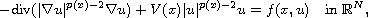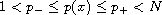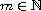Electron. J. Differential Equations, Vol. 2018 (2018), No. 31, pp. 1-20.

### Solutions for p(x)-Laplace equations with critical frequency Xia Zhang, Chao Zhang, Huimin Gao

Abstract:where. We study this equation with the potentials being zero. By using variational method, we obtain the existence of nonnegative solutions. Moreover, if f(x,t) is odd in t, for anywe derive m pairs of nontrivial solutions.Xia Zhang Department of Mathematics Harbin Institute of Technology, China email: zhangxia@hit.edu.cn Chao Zhang Department of Mathematics Harbin Institute of Technology, China email: czhangmath@hit.edu.cn Huimin Gao Department of Mathematics Harbin Institute of Technology, China email: 1576055240@qq.com